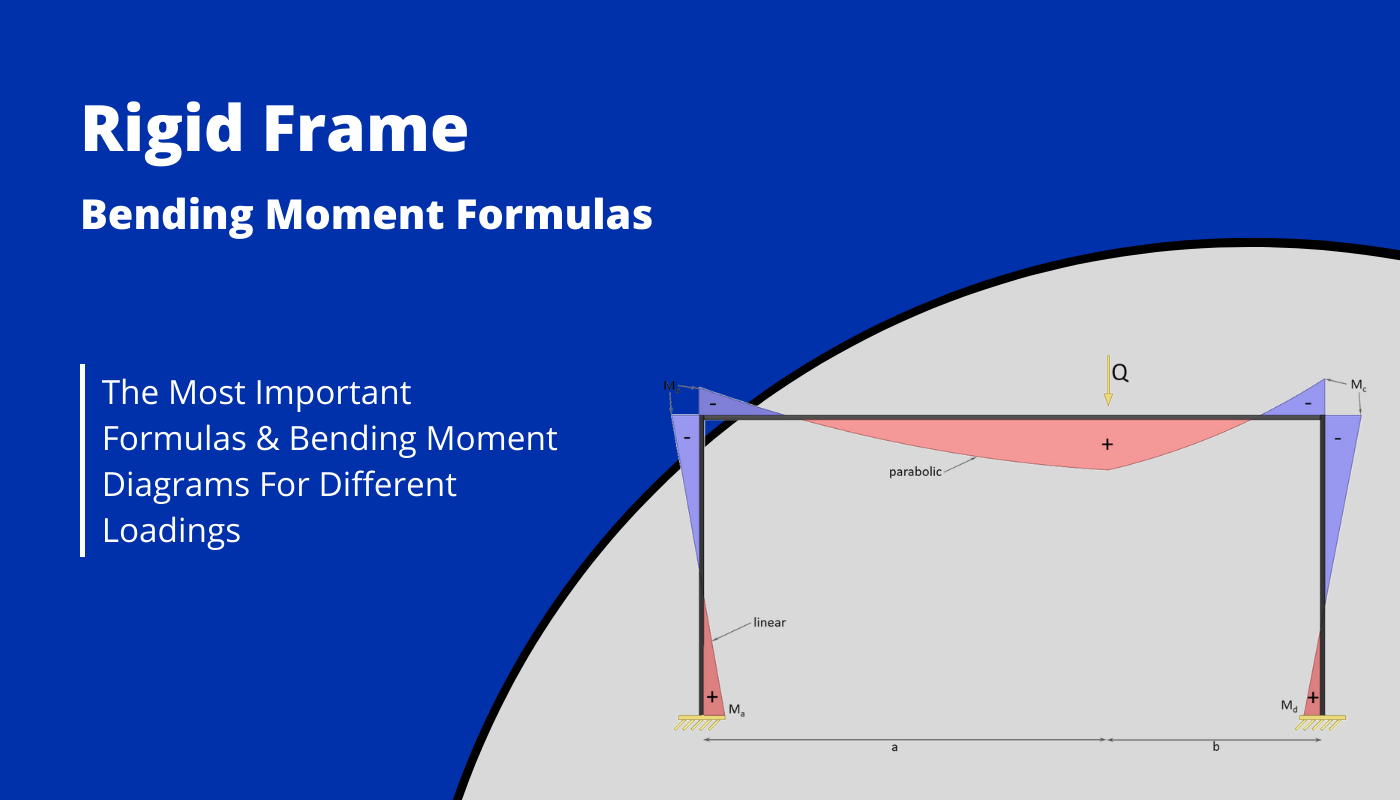# Rigid Frame Structure: Moment formulas – Different loadsLast updated: November 14th, 2022

Calculating bending moments in structural elements – in this case rigid frames with two fixed supports – for different loading scenarios, is probably one of the things in structural engineering that we do throughout our studies and also careers later on.

While it’s very important to know how to derive and calculate reaction and internal forces, the further we get in our studies, the more we can use frame moment formulas.

In earlier articles, we already covered moment and shear force formulas and diagrams for:

Before we start, some boundary conditions are set, such as that

• the 2 columns have the same Moment of inertia $I_1$ and a length of h
• the top beam has the Moment of inertia $I_2$ and a length of l
• the parameter k is defined as $\frac{I_2}{I_1} \cdot \frac{h}{l}$.

## 1. Uniformly distributed line load (UDL) on beam

#### Moment (support a & d)

$M_a = M_d = \frac{q \cdot l^2}{12\cdot (k+2)}$

#### Moment (b & c)

$M_b = M_c = -\frac{q \cdot l^2}{6\cdot (k+2)}$

## 2. Uniformly distributed load (UDL) on both columns

#### Moment (support a & d)

$M_a = M_d = – \frac{1}{12} \cdot q \cdot h^2 \cdot \frac{k+3}{k+2}$

#### Moment (b & c)

$M_b = M_c = – \frac{1}{12} \cdot q \cdot h^2 \cdot \frac{k}{k+2}$

## 3. Uniformly distributed load (UDL) on 1 column

#### Moment (support a)

$M_a = \frac{1}{24} \cdot q \cdot h^2 \cdot (\frac{5k+9}{k+2}-\frac{12k}{6k+1})$

#### Moment b

$M_b = – \frac{1}{24} \cdot q \cdot h^2 \cdot (\frac{12k}{6k+1}+\frac{k}{k+2})$

#### Moment c

$M_c = \frac{1}{24} \cdot q \cdot h^2 \cdot (\frac{12k}{6k+1}-\frac{k}{k+2})$

#### Moment (support d)

$M_d = – \frac{1}{24} \cdot q \cdot h^2 \cdot (12 – \frac{5k+9}{k+2} – \frac{12k}{6k+1})$

## 4. Point load on beam

#### Moment (support a)

$M_a = \frac{Q\cdot a \cdot b}{2\cdot l} \cdot \frac{5k-1+2\cdot(k+2)\cdot \frac{a}{l}}{(k+2)\cdot(6k+1)}$

#### Moment b

$M_b = – \frac{Q\cdot a \cdot b}{2\cdot l} \cdot \frac{13k+4-2\cdot(k+2)\cdot \frac{a}{l}}{(k+2)\cdot(6k+1)}$

#### Moment c

$M_c = – \frac{Q\cdot a \cdot b}{2\cdot l} \cdot \frac{11k+2\cdot(k+2)\cdot \frac{a}{l}}{(k+2)\cdot(6k+1)}$

#### Moment (support d)

$M_d = \frac{Q\cdot a \cdot b}{2\cdot l} \cdot \frac{7k+3-2\cdot(k+2)\cdot \frac{a}{l}}{(k+2)\cdot(6k+1)}$

## 5. Point load on Column

#### Moment (support a & d)

$M_a = -M_d = – \frac{1}{2} \cdot Q \cdot h \cdot \frac{3k+1}{6k+1}$

#### Moment (b & c)

$M_b = -M_c = \frac{1}{2} \cdot Q \cdot h \cdot \frac{3k}{6k+1}$

If you are new to structural design, then check out our design tutorials where you can learn how to use the calculated bending moments and shear forces to design structural elements such as

Do you miss any formulas for this rigid frame that we forgot in this article? Let us know in the comments✍️